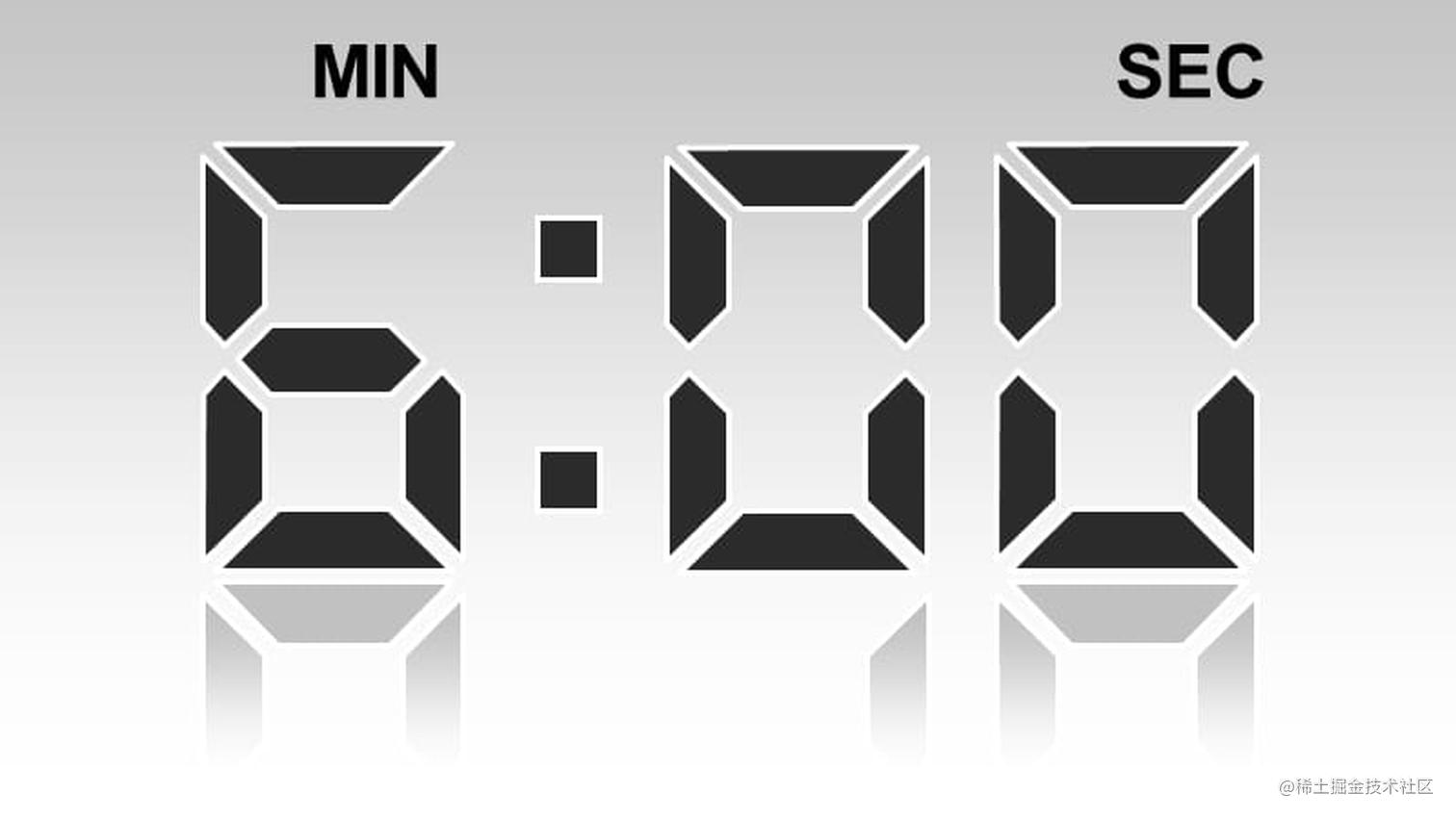# 六分钟带你学会 react 中的 useMemo## 概念

`react` 中是通过一次次的 `re-render` （重新渲染）保持我们的值及时的更新到页面上的，每次重新渲染都是一次快照，可以把它想象成一张张的照片，在某个时刻它应该是什么样子的

## useMemo

• 把创建函数和依赖数组项作为参数传入 `useMemo`，它仅仅会在数组依赖项中的值改变时才会重新计算值
• 这种优化有助于避免在每次渲染时都进行高开销的计算
• useMemo 的函数在渲染期间执行，所以不该在此期间做的操作请去除
• 如果没有提供依赖数据，每次都会重新计算值，相当于没有优化了

## 栗子

### 筛选偶数

``````import React, { useState } from 'react';

export default () => {
const [count, setCount] = useState(100);

const arr = [];
for (let i = 0; i < count; i++) {
if (i % 2 === 0) {
arr.push(i);
}
}

return (
<>
<form>
<input
type="number"
value={count}
onChange={(event) => {
// 设置最大值为 100000
let num = Math.min(100_000, Number(event.target.value));
setCount(num);
}}
/>
</form>
<p>
有{arr.length}偶数在 0 到 {count} 之间:<span>{arr.join(', ')}</span>
</p>
</>
);
};

### 每秒获取时间刷新页面

``````import React, { useState, useEffect } from 'react';

export default () => {
const [count, setCount] = useState(100);
const [curTime, setCurTime] = useState('');

const useTime = () => {
useEffect(() => {
const intervalId = window.setInterval(() => {
let time = new Date();
setCurTime(time.toLocaleString());
}, 1000);

return () => {
window.clearInterval(intervalId);
};
}, []);

return curTime;
};
const time = useTime();

const arr = [];
for (let i = 0; i < count; i++) {
if (i % 2 === 0) {
arr.push(i);
}
}

return (
<>
<form>
<div>{time}</div>
<input
type="number"
value={count}
onChange={(event) => {
// 设置最大值为 100000
let num = Math.min(100_000, Number(event.target.value));
setCount(num);
}}
/>
</form>
<p>
有{arr.length}偶数在 0 到 {count} 之间:<span>{arr.join(', ')}</span>
</p>
</>
);
};

### 如何优化

``````const arr = useMemo(() => {
const temp = [];
for (let i = 0; i < count; i++) {
if (i % 2 === 0) {
temp.push(i);
}
}
return temp;
}, [count]);

## useCallback、React.memo

• React.memo：当其作用于函数式组件并且作为子组件时, 每次父组件更新后, 会浅对比传来的 props 是否变化, 若没变化, 则子组件不更新
• useCallback：作用和 useMemo 一致，返回一个函数

``````// 父组件代码：一个计时器每秒更新时间，父组件每秒不停的 re-render，改变 count 值的 onCountChange 函数传入子组件
import React, { useState, useEffect, useCallback } from 'react';
import Child from './child';

export default () => {
const [count, setCount] = useState(100);
const [curTime, setCurTime] = useState('');

const useTime = () => {
useEffect(() => {
const intervalId = window.setInterval(() => {
let time = new Date();
setCurTime(time.toLocaleString());
}, 1000);

return () => {
window.clearInterval(intervalId);
};
}, []);

return curTime;
};
const time = useTime();
const onCountChange = () => {
setCount((count) => count + 100);
};
console.log('re-render-father');

return (
<>
<div>{time}</div>
<div>{count}</div>
<Child onCountChange={onCountChange} />
</>
);
};

// 子组件代码，接收 onCountChange 函数，并且用 React.memo 包裹函数
import React from 'react';

export default React.memo((props: any) => {
const { onCountChange } = props;
console.log('re-render-child');

return (
<>
<div
onClick={() => {
onCountChange();
}}
>
加100
</div>
</>
);
});

• 现象：父组件和子组件都会不停的 re-render
• 我子组件加了 React.memo ，虽然父组件因为计时器在不停的 re-render，但是我每次传入 onCountChange 的函数都是一样的啊，不是说比较 props 没变就不会 re-render 吗？？？为啥也会不停的 re-render 呢

``````const onCountChange = useCallback(() => {
setCount((count) => count + 100);
}, []);

``````const onCountChange = useMemo(() => {
return () => {
setCount((count) => count + 100);
};
}, []);

## 总结

• useMemo 优化我们代码的手段，可以帮助我们使用缓存的值或者函数减少重复计算或者重复的渲染，优化性能
• useCallback 作用和 useMemo 基本一致
• useMemo 或者 useCallback 在 React.memo 因为 props 中因为`引用值`而失效的时候可以帮助使用缓存值（同一个引用），从而在浅比较的时候不会 re-render